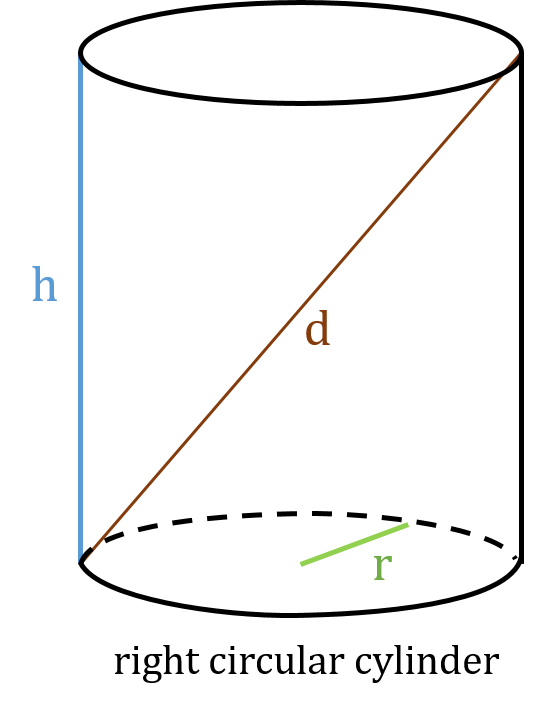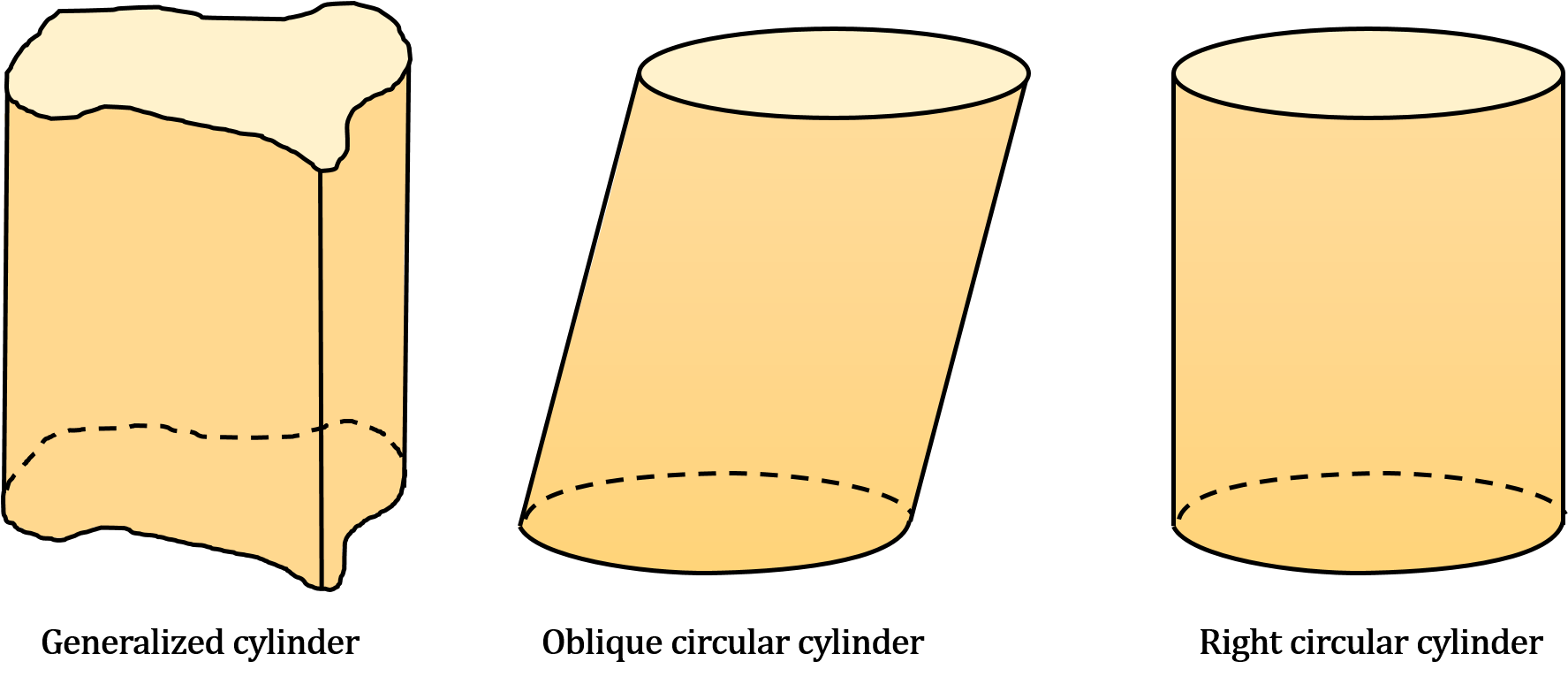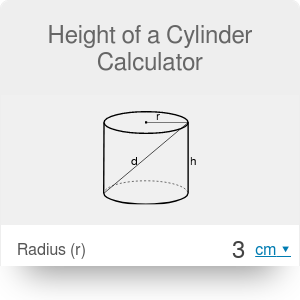in
Volume
cu in
Diagonal (d)
in
Surface areas
Base surface area
in²
Lateral surface area
in²
Total surface area
in²
Height
Height (h)
in

# Height of a Cylinder Calculator

By Dominik Czernia, PhD candidate

This height of a cylinder calculator quickly finds the height of a right circular cylinder in ten different ways. Do you wonder how to find the height of a cylinder? Just choose which two of the parameters you know, enter specified values and compute the height.

Keep reading if you want to learn what are possible height of a cylinder formulas. In most cases, you can estimate it knowing only two of the below quantities:• volume,
• longest diagonal,
• base surface area,
• lateral surface area,
• or total surface area.

Our height of a cylinder calculator is a handy tool dedicated to the right circular cylinder. This type of cylinders consists of two congruent circles (called bases). They lie precisely one above the other, and that's why we call it a right cylinder. On the other hand, if one of the bases is shifted, then a cylinder is oblique. The term circular is more obvious - bases have the form of circles. You should remember that the word cylinder may correspond to the different shapes (generalized cylinder), but we usually have in mind the right circular cylinder.

The calculator on this site was prepared to answer the question how to find the height of a cylinder. If you want to estimate another parameters, though, check out our right circular cylinder calculator right now!## What's the height of a cylinder formula?

There are five basic equations which completely describe the cylinder with given radius `r` and height `h`:

1. Volume of a cylinder: `V = π * r² * h`,
2. Base surface area of a cylinder: `A_b = 2 * π * r²`,
3. Lateral surface area of a cylinder: `A_l = 2 * π * r * h`,
4. Total surface area of a cylinder: `A = A_b + A_l`,
5. Longest diagonal of a cylinder: `d² = 4 * r² + h²`.

Sometimes, however, we have a different set of parameters. With this height of a cylinder calculator you can now quickly use ten various height of a cylinder formulas which can be derived directly from the above equations:

1. Given radius and volume: `h = V / (π * r²)`,
2. Given radius and lateral area: `h = A_l / (2 * π * r)`,
3. Given radius and total area: `h = (A - 2 * π * r²) / (2 * π * r)`,
4. Given radius and longest diagonal: `h = √(d² - 4 * r²)`,
5. Given volume and base area: `h = 2 * V / A_b`,
6. Given volume and lateral area: `h = A_l² / (4 * π * V)`,
7. Given base area and lateral area: `h = √(A_l² / (2 * π * A_b))`,
8. Given base area and total area: `h = (A - A_b) / √(2 * A_b * π)`,
9. Given base area and diagonal: `h = √(d² - 2 * A_b / π)`,
10. Given lateral area and total area: `h = A_l / √(2 * π * (A - A_l))`.

Are you interested in right circular cylinder calculations? You certainly need to check out cylinder volume calculator and surface area of a cylinder calculator!

## How to find the height of a cylinder?

The height of a cylinder calculator is very easy to use in a wide range of different problems. Sometimes you will know the volume and the base area of a cylinder, and you won't know the height of it. Another time you will have only surface areas specified. If you ever face that kind of problems, use this calculator to estimate height in three simple steps:

1. Determine which parameters of a cylinder you know. You need to have at least two of them.
2. Enter values of the selected quantities.
3. Read out the result of the calculations.

Remember that with our height of a cylinder calculator you can choose units of every parameter you want. Be sure to check out the length and the volume conversion tools as well. They can be very helpful in many calculations too!

Dominik Czernia, PhD candidate

## Get the widget!

Height of a Cylinder Calculator can be embedded on your website to enrich the content you wrote and make it easier for your visitors to understand your message.

It is free, awesome and will keep people coming back!